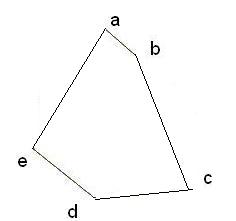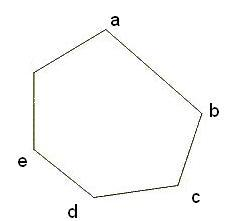## Polygon Calculator

Calculate the various properties of Area of Polygon for given values.
 Area of Polygon [Using length of a side] : [ ((side)²×N)/(4Tan(π/N)) ] Enter the length = Enter the Number of Sides = Area of Polygon =

 Area of Polygon [Using radius (circumradius)] : [ ½×R²×Sin(2π/N) ] Enter the Radius = Enter the Number of Sides = Area of Polygon =

 Area of Polygon [Using apothem (inradius)] : [ A²×N×Tan(π/N) ][ Apothem(A) = R×Cos(π/N) ] Enter the Radius = Enter the Number of Sides = Area of Polygon =

 Area of Polygon [Using apothem and length of a side] : [ (A×P)/2 ][ Apothem(A) = side/(2×Tan(π/N)) ] Enter the Length = Enter the Number of Sides = Area of Polygon =

 Perimeter of Polygon:[ N×(side) ] Enter the Length = Enter the Number of Sides = Perimeter of Polygon =

 Polygons Type Definition Shape Regular polygons The polygon having all sides and interior angles of the same size.Irregular polygons Every side and angle may be of different size.Convex polygons Every interior angles are < 180° and every vertices 'point outwards' away from the interior. The regular polygons are always convex.Concave polygons One or more interior angles > 180° and some vertices push 'inwards' towards the interior of the polygon.Crossed polygons The The polygon where any or more sides are crossing back over another side means forming multiple smaller polygons.